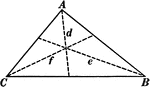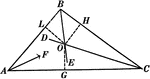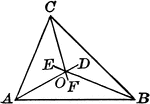### Centers of Equilateral Triangle

Illustration of an equilateral triangle that shows both the centroid (where the medians of the sides…### Angle Bisectors In A Triangle

Illustration showing the three angle bisectors in a triangle.### Angle Bisectors In A Triangle

Illustration used to prove "The bisectors of the angles of a triangle are concurrent in a point which…### Incenter of Triangle

Illustration to show angle bisectors in a triangle. It is known as the incenter.